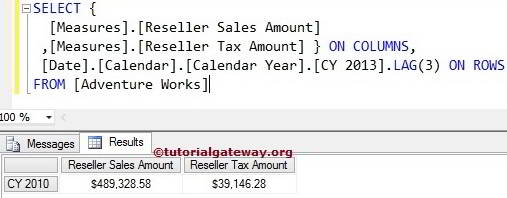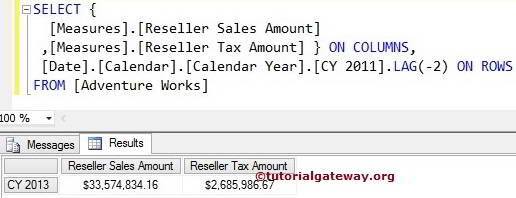# MDX LAG Function

The MDX Lag function is used to navigate between same level members and returns the members at the specified position. For example, If you want to navigate to a particular member, then we can use this MDX Lag function.

## MDX Lag Function Syntax

The basic syntax of the Lag Function in Multidimensional Expression is as shown below:

`Member_Expression.LAG (Member_Position)`

Member_Expression: Any Multidimensional Expression that returns a valid Member.

Member_Position: Please specify the position of a member you want to Navigate.

• If we use Zero as the Member_Position, then MDX Lag Function will write the same Member_Expression that we mentioned before Lag function.
• If we use Negative Value as the Member_Position, then Lag Function will move forward to a specified value and returns the Member_Expression at that position.
• If we use Positive Value as the Member_Position, the Lag Function will move Backwards to the specified value and returns the Member_Expression at that position.

The MDX Lag Function is used to navigate between the same Members level. For example, If we mention a City member as Member_Expression, the Lag function will navigate between the remaining Cities. It will not go Level Up (State – Province) or Level down (Postal Code).

How to write a Lag function to navigate both forward and backward with examples? For this, we are going to use the below-shown data.

## MDX Lag Function with Zero

In this example, we will show you, What happens when we use Zero value for the Lag Function. The following query will return the Reseller Sales amount and Tax amount of the Calendar Year 2013 itself.

TIP: In MDX, Lead Function with Positive Value is Equal to Lag with Negative value.

```SELECT {
[Measures].[Reseller Sales Amount]
,[Measures].[Reseller Tax Amount] } ON COLUMNS,
[Date].[Calendar].[Calendar Year].[CY 2013].LAG(0) ON ROWS

## MDX Lag Function with Positive Value

This example shows what happens when we use a Positive integer value in Lag Function. The following query will return the Reseller Sales amount and Tax amount of the Calendar Year 2010 because the Lag function with Positive value (3) will move back 3 years from the given year (2013 – 3 = 2010)

```SELECT {
[Measures].[Reseller Sales Amount]
,[Measures].[Reseller Tax Amount] } ON COLUMNS,
[Date].[Calendar].[Calendar Year].[CY 2013].LAG(3) ON ROWS## MDX Lag Function with Negative Value

In this example, we show you, What happens when we use the Negative integer value for the Lag Function. The following MDX query returns the Reseller Sales amount and Tax amount of the Calendar Year 2013. Because Lag function with Negative value (-2) will move forward 2 years from the given year (2011 + 2 = 2013)

```SELECT {
[Measures].[Reseller Sales Amount]
,[Measures].[Reseller Tax Amount] } ON COLUMNS,
[Date].[Calendar].[Calendar Year].[CY 2011].LAG(-2) ON ROWS## Finding Range Using MDX Lag Function

How to find the Range using the Lag function?. The following query will return the Reseller Sales amount and Tax amount from Calendar Year 2010 to 2013 because we used the range symbol (:) between [CY 2013] and [CY 2013].LAG (3).

```SELECT {
[Measures].[Reseller Sales Amount]
,[Measures].[Reseller Tax Amount] } ON COLUMNS,
[Date].[Calendar].[Calendar Year].[CY 2013]:
[Date].[Calendar].[Calendar Year].[CY 2013].LAG(3) ON ROWS